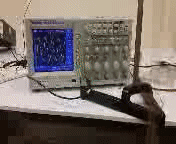# RLC circuit resonance with an oscillating inductor (Jr IS Guest blog by Kyle McNickle)The purpose of my experiment was to analyze whether the behavior of an RLC (Resistor, Inductor, Capacitor) circuit is noticeably affected by replacing the inductor with an oscillating spring. Common inductors take the form of solenoids which are helical coils of wire that are wrapped around a core. This core can be made of different materials, but some of the more common are ferromagnetic materials like iron, cobalt, or nickel. The core can also just be air, which is similar to the model that the spring mimics.

When a current starts to run through an inductor, the inductor resists the current. After a brief period of time, the inductor will no longer resist the current and the system is in equilibrium. If the current changes, however, the system will no longer be in equilibrium and the inductor will once again try to resist the change. In order for the inductor to resist the change, there must be some sort of force that it applies to the current. It turns out that this force is exerted through the use of a magnetic field acting on the current. Therefore, we say that if the current changes, it induces a magnetic field that resists the change in the current. This is a reciprocal relationship, too. If instead, the magnetic flux (the amount of magnetic field flowing through the area of the inductor) changes, then a current will be induced that opposes the changing flux.

By exploiting this trait of inductors, my hope was to see significant change in the output voltage of my circuit. For an RLC circuit, the output voltage is dependent on the frequency that is driving the circuit. For certain frequencies, the output voltage will be approximately zero, whereas for other frequencies, the output voltage will be immense. The curve on a graph of output voltage as a function of frequency is called the resonance curve and the maxima of this curve is referred to as the resonant frequency. The width of the curve as well as the location of the resonant frequency are dictated by the inductance in the circuit. The inductance of an inductor is dependent on some of its geometrical properties such as its length and radius. Therefore, with a spring, the geometry can be altered and inductance can be actively changed as the circuit is running.

When I placed the spring in the circuit, I was able to observe the resonant frequency shifting to different values by stretching or compressing it. For one trial, a mass was suspended from the spring and the mass/spring system was made to oscillate. During the period of oscillation, the inductance of the spring was constantly changing which meant the inductor was continuously responding to changes in current and magnetic flux and resisting them accordingly. While observing the electrical, sinusoidal signal passing through the circuit on an oscilloscope, I could actually see the amplitude of the signal changing corresponding to movement of the spring. See attached video in order to watch this phenomenon.

This entry was posted in Uncategorized. Bookmark the permalink.